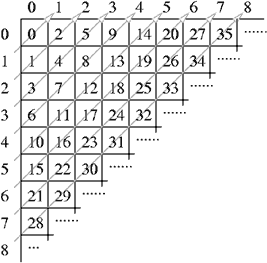Online JudgeProblem SetAuthorsOnline ContestsUser
Web Board
F.A.Qs
Statistical Charts
Problems
Submit Problem
Online Status
Prob.ID:
Register
Authors ranklist
Current Contest
Past Contests
Scheduled Contests
Award Contest
Register
Language:
Polygon Encoder
 Time Limit: 1000MS Memory Limit: 65536K Total Submissions: 704 Accepted: 120

Description

Imagine an infinite table with rows and columns numbered using the natural numbers. The following figure shows a procedure to traverse such a table assigning a consecutive natural number to each table cell:This enumeration of cells can be used to represent complex data types using natural numbers:

• A pair of natural numbers (i, j) is represented by the number corresponding to the cell in row i and column j. For instance, the pair (3, 2) is represented by the natural number 17; this fact is noted by P2(3, 2) = 17.

• The pair representation can be used to represent n-tuples. A triplet (a, b, c) is represented by P2(a, P2(b, c)). A 4-tuple (a, b, c, d) is represented by P2(a, P2(b, P2(c, d))). This procedure can be generalized for an arbitrary n:

Pn(a1, …, an) = P2(a1, Pn−1(a2, …, an)),

where Pn denotes the n-tuple representation function, n ≥ 2. For example P3(2, 0, 1) = 12.

• A list of arbitrary length ‹a1, …, an› is represented by

L(‹a1, …, an›) = P2(n, Pn(a1, …, an)).

For example, L(‹0, 1›) = 12.

The Association of Convex Makers (ACM) uses this clever enumeration scheme in a polygon representation system. The system can represent a polygon, defined by integer coordinates, using a natural number as follows: given a polygon defined by a vertex sequence ‹(x1, y1), …, (xn, yn)› assign the natural number:

L(‹P2(x1, y1), …, P2(xn, yn)›).

ACM needs a program that, given a natural numbers that represents a polygon, calculates the area of the polygon. It is guaranteed that the given polygon is a simple one, i.e. its sides do not intersect.

As an example of the problem, the triangle with vertices at `(1,1)`, ` (2,0)` and ` (0,0)` is codified with the number `2141`. The area of this triangle is `1`.

Input

The input consists of several test cases. Each test case is given in a single line of the input by a natural number representing a polygon. The end of the test cases is indicated with `*`.

Output

One line per test case, preserving the input order. Each output line contains a decimal number telling the area of the corresponding encoded polygon. Areas must be printed with 1 decimal place, truncating less significative decimal places.

Sample Input

```2141
206
157895330
*```

Sample Output

```1.0
0.5
1.0```

Source

[Submit]   [Go Back]   [Status]   [Discuss]Home PageGo BackTo top